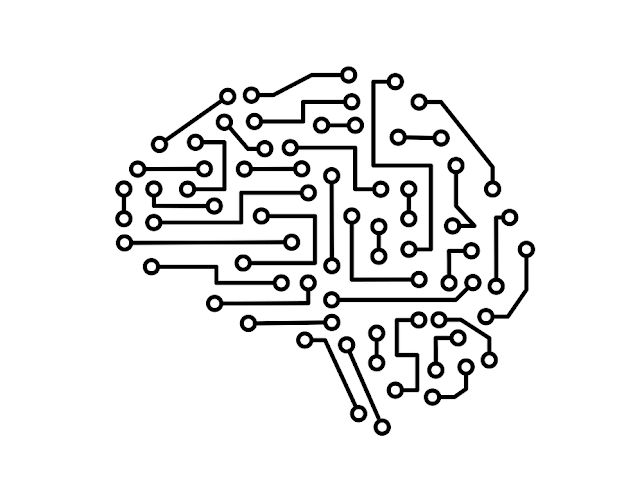Distance metrics are an integral part of both supervised and unsupervised machine learning. They are used primarily to calculate the distance between data points. The use of a useful distance metric enhances the performance of the machine learning model.

Four types of distance metrics are used in machine learning: Euclidean Distance, Manhattan Distance, Minkowski Distance, and Hamming Distance. This article explores the use of Manhattan Distance in Machine Learning. So, what is Manhattan Distance?

## Manhattan Distance – Explained

Simply put, Manhattan Distance is the distance between two real-valued points or vectors. It is the sum of differences between points. The distance is measured along axes at right angles. Manhattan Distance between two points (x1, y1) and (x2, y2) is: |x1 – x2| + |y1 – y2|.

Computation of Manhattan Distance forms part of Taxicab Geometry used for various problems since the 18th century. This approach of geometry is often called the LASSO approach.

## Representation

The Manhattan Distance, also called the city block distance, or taxicab geometry, is represented using a grid. Users assume the entire grid is a city block and each cell is a building. If a person wants to travel from Point A to Point B in the grid, they will choose between two or both paths, each with its turns.

In a situation like this, The Manhattan Distance Metric can be used to calculate the distance traveled. It helps calculate the shortest route a taxicab could take between two city blocks.

## Manhattan Distance in Machine Learning Models

Distance measures play a significant role in machine learning when learning with Fernstudium-Infomaterial. The Manhattan Distance is most useful to vectors that relate to objects on a unified grid. It is typically used in scenarios where dimensions are not comparable.

The gap between the two data points is the sum of variations between their components. The Manhattan Distance Metric is preferred over the Euclidean Distance Metric in situations where the data dimension increases. This follows from the concept called "the curse of dimensionality.”

## Other Distance Metrics Used in Machine Learning

Now that you know the answer to the question “What is Manhattan Distance?” here's a look at the other distance metrics used in Machine Learning Models. The other distance metrics used in machine learning are listed below:

Minkowski Distance: It is a generalized distance metric used inside a normed vector space. It is used to evaluate the similarity of distances between two or more vectors. This metric is mostly used in machine learning to determine the similarity of size.

Euclidean Distance: It is a popular distance metric used to measure the similarity between observations. This metric is used when there are fewer dimensions.

Hamming Distance: It is used to analyze the similarity between two lines of the same length. It focuses on counting which set of figures are different and which are the same. It is applied mainly to problems of error correction or comparison of opposing strings orbits.

## Application in Machine Learning Models

All machine learning models, whether supervised or unsupervised, use distance metrics. Distance Metrics are used in machine learning to make data-based decisions and to assess data patterns.

Application of the right distance metric will enhance the machine learning algorithm's functioning and result in faster, more accurate results. The right choice and systemic employment of the proper distance metric will improve the speed of data classification, clustering, and information retrieval.